DIFFERENTIATION USING THE QUOTIENT RULE

The following problems require the use of the quotient rule. In the following discussion and solutions the derivative of a function h(x) will be denoted by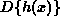or h'(x) . The quotient rule is a formal rule for differentiating problems where one function is divided by another. It follows from the limit definition of derivative and is given by.

Remember the rule in the following way. Always start with the ``bottom'' function and end with the ``bottom'' function squared. Note that the numerator of the quotient rule is identical to the ordinary product rule except that subtraction replaces addition. In the list of problems which follows, most problems are average and a few are somewhat challenging.

• PROBLEM 1 : Differentiate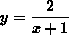.

• PROBLEM 2 : Differentiate.

• PROBLEM 3 : Differentiate.

• PROBLEM 4 : Differentiate.

• PROBLEM 5 : Differentiate.

• PROBLEM 6 : Differentiate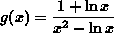.

• PROBLEM 7 : Differentiate.

Some of the following problems require use of the chain rule.

• PROBLEM 8 : Differentiate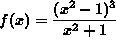.

• PROBLEM 9 : Consider the function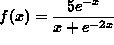. Evaluate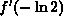.

• PROBLEM 10 : Differentiate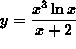.

• PROBLEM 11 : Differentiate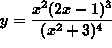.

• PROBLEM 12 : Differentiate.

• PROBLEM 13 : Differentiate.

• PROBLEM 14 : Differentiate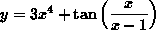.

• PROBLEM 15 : Differentiate• PROBLEM 16 : Find an equation of the line tangent to the graph of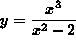at x=-1 .

• PROBLEM 17 : Find an equation of the line tangent to the graph ofat.

• PROBLEM 18 : Consider the function. Solve f'(x) = 0 for x . Solve f''(x) = 0 for x .

• PROBLEM 19 : Find all points (x, y) on the graph ofwhere tangent lines are perpendicular to the line 8x+2y = 1 .